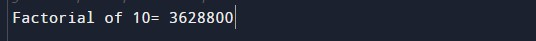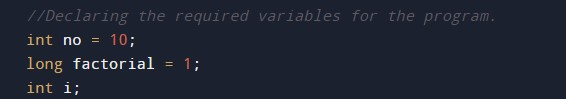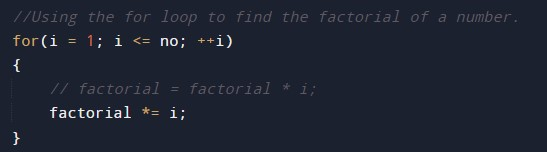# Java Program to Find Factorial of a Number

In this tutorial you will learn about the Java Program to Find Factorial of a Number and its application with practical example.

## Java Program to Find Factorial of a Number

In this tutorial, we will learn to create a Java program that will Find Factorial of a Number using Java programming.

## Prerequisites

Before starting with this tutorial we assume that you are best aware of the following Java programming topics:

• Operators in Java Programming.
• Basic Input and Output function in Java Programming.
• Basic Java programming.
• Conditional Statements in Java programming.
• Arithmetic operations in Java Programming.

## What is factorial?

The factorial means the product of the input number and its below integer value up to 1.

## Program to Calculate Factorial:-

In today’s tutorial, we will learn to create a program that will find the factorial of a number. First, we will take a number in input from the user. Then we will find the factorial using the for-loop. At last, we will print the factorial of the given number to the user.

## Output:-In the above program, we have first initialized the required variable.• no = it will hold the integer value.
• i = it will hold the integer value.
• factorial = it will hold the integer value.
Defining the input for the program.Finding the factorial.In this tutorial we have learn about the Java Program to Find Factorial of a Number and its application with practical example. I hope you will like this tutorial.